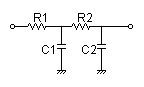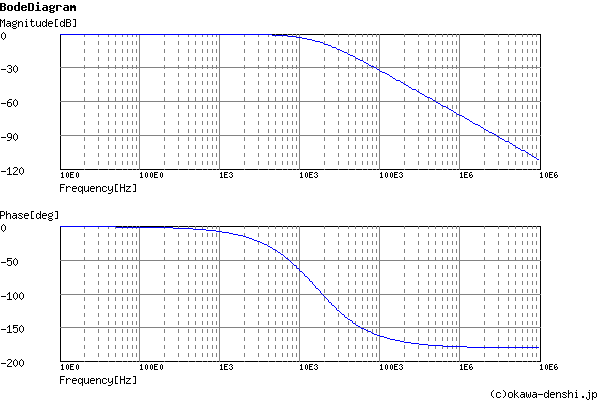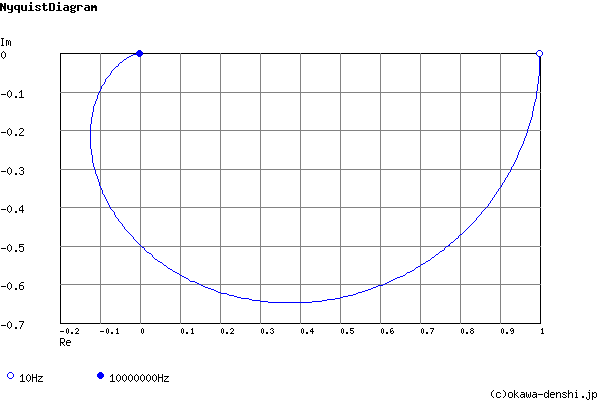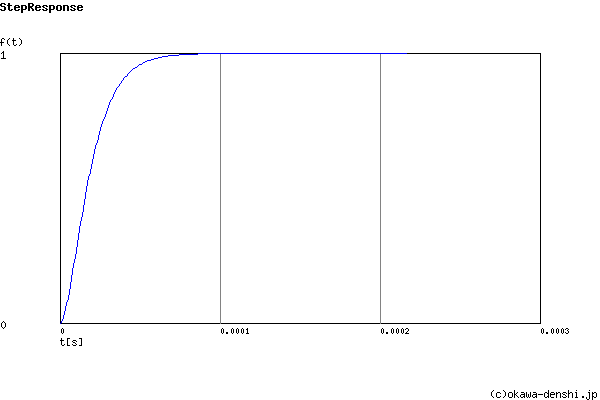# (Sample) 2nd order CR Low-pass Filter Design Tool - Result -

Calculated the transfer function for 2nd order CR Low-pass filter, displayed on graphs, showing Bode diagram, Nyquist diagram, Impulse response and Step response.

## 2nd order CR filter

 Vi(s)→→Vo(s)
(Sample)Transfer Function:
 G(s)= 10000000000 s2+201000s+10000000000

#### Center frequency

fc = 15915.4943092[Hz]

#### Q factor

Q = 0.497512437811

ζ = 1.005

#### Pole(s)

p = -14401.5341549[Hz]
|p|= 14401.5341549[Hz]
p = -17588.6094066[Hz]
|p|= 17588.6094066[Hz]

#### Phase margin

pm= NAN[deg] (f =0[Hz])

#### Overshoot (in absolute value)

The peak of transient waveform is not detected.

#### Final value of the step response (on the condition that the system converged when t goes to infinity)

g(∞) = 1

 R1=Ω C1=F R2=Ω C2=F
p:pico, n:nano, u:micro, k:kilo, M:mega

### Frequency analysis

Bode diagram
Phase  Group delay
Nyquist diagram
Pole, zero
Phase margin
Oscillation analysis
Analysis on frequency range:
f1=∼f2=[Hz] (optional)

### Transient analysis

Step response
Impulse response
Overshoot
Final value of the step response
Analysis on time range:
0∼[sec] (optional)

# Frequency analysis# Transient analysis